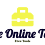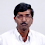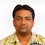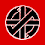### Plant Fence Pot - Math Puzzle

This is a very tricky math puzzle. Look closely on each of the clues and try to solve.

The correct answer with explanation is given below.

Explanation
Equation 1: 15 + 15 + 15 = 45  (So, the value of Plant = 15 ; Also note that in this equation the Plant has 15 leaves)
Equation 2: 15 + 2 + 2 = 19   (So, the value of Fence = 2)
Equation 3: 10 + (10 + 2) + 10 = 32   (The value of Pot = 10 ; Also, in this equation, there is a pot hiding in the back of the Fence)
Equation 4: (21 x 21) x (2 + 2) + (10 + 10)   (In this equation, there are 21 leaves for the plant & there is a square symbol above the plant ; 2 Fences (one in front of other) ; Also 2 Pots. If you look closely at top of the first pot, you can see a second pot hiding at the back of the first pot)

Applying BODMAS rule:

=> (441 x 4) + 20
=> 1764 + 20
=> 1784

1.Equation 1 plant with pot 15 .so without pot plant value is only 5 last there is plant without pot so how it become 22 pls clarify this

1.Is not like that plant has 15 leaves and in last there is 21 leaves pot is necessary ( my English is bad) 😅

2.Wrong explanation... the 15 leavs plant value with pot is 15 , without pot is 5, pot value is 10, so when 21 leaves Plant comes without pot then its value is 21 -10 = 11, so 11 square is 121 , now 121 × 4 +20 = 504

3.Then,who told you the value of plant is 21 on the besis of leavs???

2.7X7x4+20=216

1.2.if u agree with her answer as 100% correct..plsss explain fully with solved equation..

3.Explanation
Equation 1: 15 + 15 + 15 = 45 (So, the value of Plant + pot = 15 ; Also note that in this equation the Plant has 15 leaves)
Equation 2: 15 + 2 + 2 = 19 (So, the value of Fence = 2)
Equation 3: 10 + (10 + 2) + 10 = 32 (The value of Pot = 10 ; Also, in this equation, there is a pot hiding in the back of the Fence)
Since the value of pot is 10, the value of plant alone in equation 1 is 15-10 = 5. Since there are 15 leaves the value of one leaf is 5/15 = 1/3
Equation 4: (7 x 7) x (2 + 2) + (10 + 10) (In this equation, there are 21 leaves for the plant and hence its value is 21 x 1/3 = 7 & there is a square symbol above the plant ; 2 Fences (one in front of other) ; Also 2 Pots. If you look closely at top of the first pot, you can see a second pot hiding at the back of the first pot)

Applying BODMAS rule:

=> (49 x 4) + 20
=> 196 + 20
=> 216

4.Pot value is 10..so first equation leaf is 5...

Now calculate

5.Wrongly explained ... how u got 7... there when there is no pot in the last Plant... u r simply calculating on the basis of Plant leaves... 15 ÷ 3 , to get the value of 1st Plant 5. If u do it like this then it itself is 15 , if the value of pot is 10 then then without pot how it becomes 7 , even if u do it 21÷3 =7, when there is 15 leaves its value with pot is 15 including pot value of 10 , now here 21 leaves without pot become 21 -10 = 11 .

6.Explanation
Equation 1: 15 + 15 + 15 = 45 (So, the value of Plant = 15 ; Also note that in this equation the Plant has 15 leaves)
Equation 2: 15 + 2 + 2 = 19 (So, the value of Fence = 2)
Equation 3: 10 + (10 + 2) + 10 = 32 (The value of Pot = 10 ; Also, in this equation, there is a pot hiding in the back of the Fence)
Equation 4: (21 x 21) x (2 + 2) + (10 + 10) (In this equation, there are 21 leaves for the plant & there is a square symbol above the plant ; 2 Fences (one in front of other) ; Also 2 Pots. If you look closely at top of the first pot, you can see a second pot hiding at the back of the first pot)

Applying BODMAS rule:

=> (441 x 4) + 20
=> 1764 + 20
=> 1784

7.Where do you get the pot value is 10? nothing in here gives that nor can you infer it from anything.

3.7²×(2×2)+(10×2)=216

1.Can you please explain how ?

4.1.5.Given ans. Is wrong
Right Ans. Is 216

1.This comment has been removed by the author.

2.Can you please explain how you got 216?

3.Then plss can u enlighten us hw u got 216??

4.216 is wrong ... right answer is 504.. value of Plant with pot is 15 , without pot is 5, pot value is 10 , Plant has 21 leaves but without pot its value is 11 ..i.e ( 21 - 10 = 11)
Now 11 sqr ×4 +20 = 504. ....ok that is correct...

5.6.15 leaves plant without pot 5
21 leaves plant without pot = (5/15)*21 = 7, So (7x7)x4+20=216

6.i think the answer is 99 😁

1.then might be you're wrong

7.216 is the right answer.....Row 1 = 15*3 ( pot is 10 and plant is 5....15 leaves, which means 5 for a set of 3 leaves).......Row 2 = 15+2+2 .......Row 3 = 10+12+10...... Row 4 = (7*7) * 4 +20 = 216....

8.3 leaves will make a score of 1.

So,for the last row -

((21/3)^2)*4 + 2*10 = 196 + 20 = 216

9.But there is an extra branch in tree also in last equation... Then how will it solve

10.But there is an extra branch of the tree in last equation... So that will also count

11.Plant, fence, pot puzzle 216 is logical not 1784. As per 3rd eqn. pot value 10, and first eqn. plant with 15 leaves (5) and pot (10)
Last eqn. 21 leaves x 1/3 per leaf = 7*7 + 2*2 (2fence) + 20 (2 pots) = 216

12.13.14.